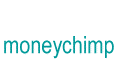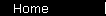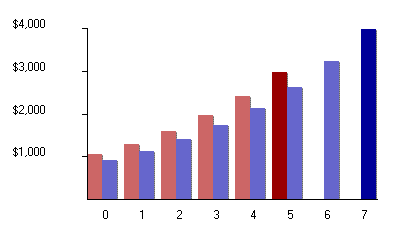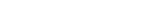# Interest on Composite Investments

In the last example we assumed you had a choice between two investments: one turned \$1000 into \$3000 in five years, and the other turned \$1000 into \$4000 in seven years. We found that these investments offered return rates of 24.57% and 21.90% respectively. But what happens if you decide to invest in both simultaneously -- that is, you put in \$2000 now, and you get \$3000 five years from now and another \$4000 seven years from now. . . . What would be the rate of return then?

By the way, the answer is not something obvious, like the average of the individual rates; that would be really great, but it's wrong. The correct rate of return has to satisfy this condition:

Whatever r is, if you use it to calculate the present values of all payouts and then add up these present values, the sum will equal your initial investment.

In other words

 1 3000(1 + r)-5 + 4000(1 + r)-7 = 2000

To understand this equation, you should be imagining two make-believe compound interest curves, each starting now at some different and unknown present value, and each growing at the same rate r. The first curve grows to \$3000 in 5 years, and stops; the second curve grows to \$4000 in 7 years, and stops. And the initial values of the two curves add up to \$2000.How do you solve equation 1 for r? Good news: you don't have to. Bad news: you can't. This is due to a famous mathematical theorem: One cannot discover a formula that solves an arbitrarily gross polynomial. Solutions exist all right (that's another famous theorem); you just aren't allowed to know exactly what they are. To get r, in general, you need to use an iterative method to find an acceptably close approximation. (If you're interested in this kind of thing, you should find a calculus book and look up Newton's method for finding roots).

Our computer-generated approximate solution to equation 1 is 23.05%. Right away, you should notice that this number is between 21.90% and 24.57%, so at least it's in the right ballpark. And if you plug 0.2305 into equation 1 for r, you find that it really is the solution, within rounding errors.

The idea used here -- of discounting future payouts back to the present, summing up these present values, and having the total equal your initial investment -- will rear its ugly head again when we calculate the yield- to- maturity of bonds.

home  |  article  |  glossary  |  calculator  |  about us  |  booksIntroductionCompound InterestMore CompoundingPresent Value / CAGRComposite InvestmentsBond YieldGeometric SeriesGrowth + ContributionsAnnuityMortgageStock ValuationBooks & Links